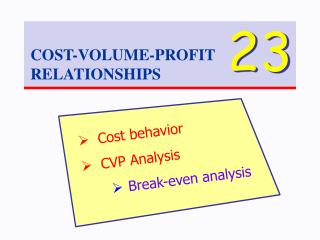# COST-VOLUME-PROFIT RELATIONSHIPS - PowerPoint PPT PresentationDownload PresentationCOST-VOLUME-PROFIT RELATIONSHIPS

COST-VOLUME-PROFIT RELATIONSHIPSDownload Presentation## COST-VOLUME-PROFIT RELATIONSHIPS

- - - - - - - - - - - - - - - - - - - - - - - - - - - E N D - - - - - - - - - - - - - - - - - - - - - - - - - - -
##### Presentation Transcript

1. 23 COST-VOLUME-PROFIT RELATIONSHIPS • Cost behavior • CVP Analysis • Break-even analysis

2. Variable Cost Cost Behavior Costs that vary in direct proportion to the level of activity. y = 1.21x R2 = 0.98 Total cost - increases with activity Per-unit cost - remains nearly constant = \$1.21 / ticket

3. Fixed Cost Costs that are unrelated to the level of activity. y = 1604 R2 = 0.00 Total cost – remains nearly constant = \$1604 / month Per-unit cost - decreases with increase in activity (Note: Per-unit fixed cost can be misleading.)

4. Variable or Fixed Cost? Some expenses have both fixed and variable components. y = 0.59x + 3,625 R2 = 0.92

5. Cost Functions An algebraic expression representing a cost as a function of fixed and variable components. Y = F + VX Y = total cost F = fixed component V = variable cost per unit X = number of units Vehicle Maintenance = \$3,625 + \$0.59 X

6. High-Low Method ( 17,700, \$13,100 ) ( 3,300, \$5,620 )

7. ( 17,700, \$13,100 ) ( 3,300, \$5,620 ) \$7,480 14,400 (1) Find the variable cost (slope). \$7,480 14,400 Variable cost: = \$0.52/unit

8. ( 17,700, \$13,100 ) ( 3,300, \$5,620 ) (2) Find the fixed cost (y-intercept). ( 17,700, \$13,100 ) Slope = \$0.52 Slope = \$0.52 Fixed cost: \$13,100 - \$0.52 x 17,700 = \$3,896 ~ \$4,000

9. Least-Squares Regression A more precise way to estimate cost functions. Use trendline function in Excel. Takes all points into account. y = 0.59x + 3,625 R2 = 0.92

10. Relevant Range Don’t use a trendline to estimate. \$8,450 \$4,100 Some expenses are fixed within a certain range.

11. Plausible Cost Functions Costs cannot be negative! Consider this a fixed cost equal to the average amount.

12. Cost-Volume-Profit Analysis An investigation of the interrelationships among: • Volume of output • Sales price • Variable costs • Fixed costs • Product mix

13. Contribution Income Statement Total Sales \$ 42,000 Less Variable Expenses 22,200 Contribution Margin \$ 19,800 Less Fixed Expenses 13,860 Net Income \$ 5,940 Per Unit \$ 350 185 \$ 165 CM: covers fixed expenses & provides profit

14. Break-Even Point Total fixed expenses Per unit contribution margin \$13,860 165 The level of sales at which the contribution margin is just enough to cover fixed expenses Break-even point = = = 84 units

15. At 84 units… Total Sales Less Variable Expenses Contribution Margin Less Fixed Expenses Net Income Total \$ 29,400 15,540 \$ 13,860 13,860 \$ 0 Per Unit \$ 350 185 \$ 165

16. Contribution Margin Ratio \$165 \$350 Per Unit Sales \$ 350 Less Variable Expenses 185 Contribution Margin \$ 165 CM Ratio = = 47.1%

17. Break-Even Point Total fixed expenses Contribution margin ratio \$13,860 .471 In sales dollars Break-even point = = = \$29,400

18. Target Net Profit How many units must be sold to earn a net income of \$8,000? x = number of units Sales - Variable - Fixed = Income 350x - 185x - 13,860 = 8,000 165x = 21,860 x = 133